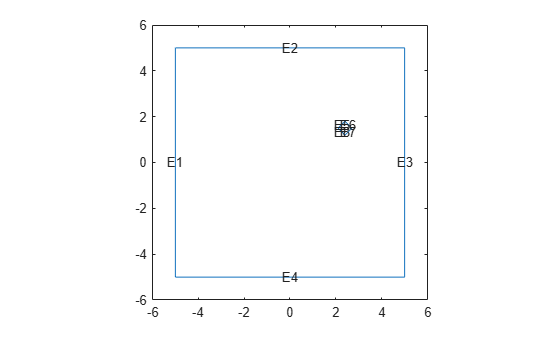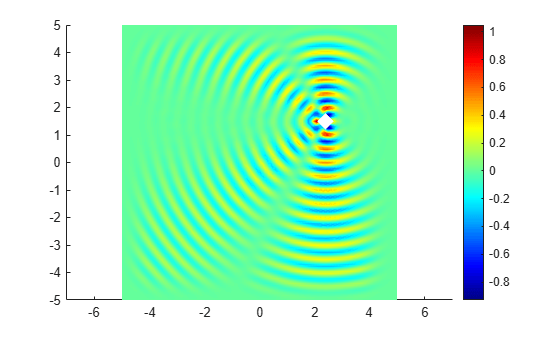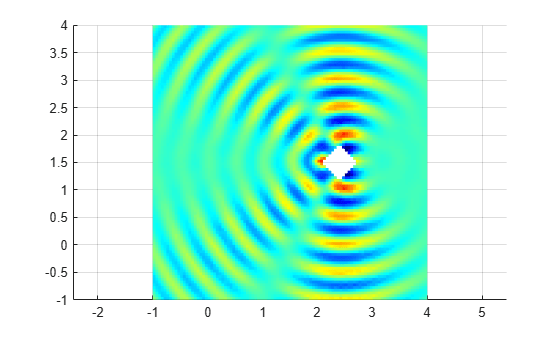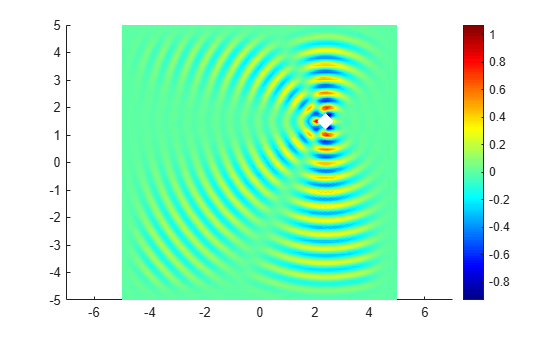Documentation

### This is machine translation

Mouseover text to see original. Click the button below to return to the English version of the page.

To view all translated materials including this page, select Country from the country navigator on the bottom of this page.

## Helmholtz's Equation on Unit Disk with Square Hole

This example shows how to solve a Helmholtz equation using the `solvepde` function.

The Helmholtz equation, an elliptic equation that is the time-independent form of the wave equation, is.

Solving this equation allows us to compute the waves reflected by a square object illuminated by incident waves that are coming from the left.

### Problem Definition

The following variables define our problem:

• `g`: A geometry specification function. For more information, see the code for `scatterg.m` and the documentation section Create Geometry Using a Geometry Function.

• `k`, `c`, `a`, `f`: The coefficients and inhomogeneous term.

```g = @scatterg; k = 60; c = 1; a = -k^2; f = 0; ```

### Create PDE Model

Create a PDE Model with a single dependent variable.

```numberOfPDE = 1; model = createpde(numberOfPDE); ```

Convert the geometry and append it to the pde model.

```geometryFromEdges(model,g); ```

### Specify PDE Coefficients

```specifyCoefficients(model,'m',0,'d',0,'c',c,'a',a,'f',f); ```

### Boundary Conditions

Plot the geometry and display the edge labels for use in the boundary condition definition.

```figure; pdegplot(model,'EdgeLabels','on'); axis equal title 'Geometry With Edge Labels Displayed'; ylim([0,1]) ```Apply the boundary conditions.

```bOuter = applyBoundaryCondition(model,'neumann','Edge',(5:8),'g',0,'q',-60i); innerBCFunc = @(loc,state)-exp(-1i*k*loc.x); bInner = applyBoundaryCondition(model,'dirichlet','Edge',(1:4),'u',innerBCFunc); ```

### Create Mesh

```generateMesh(model,'Hmax',0.02); figure pdemesh(model); axis equal ```### Solve for Complex Amplitude

The real part of the vector `u` stores an approximation to a real-valued solution of the Helmholtz equation.

```result = solvepde(model); u = result.NodalSolution; ```

### Plot FEM Solution

```figure % pdeplot(model,'XYData',real(u),'ZData',real(u),'Mesh','off'); pdeplot(model,'XYData',real(u),'Mesh','off'); colormap(jet) ```### Animate Solution to Wave Equation

Using the solution to the Helmholtz equation, construct an animation showing the corresponding solution to the time-dependent wave equation.

```figure m = 10; h = newplot; hf = h.Parent; axis tight ax = gca; ax.DataAspectRatio = [1 1 1]; axis off maxu = max(abs(u)); for j = 1:m uu = real(exp(-j*2*pi/m*sqrt(-1))*u); pdeplot(model,'XYData',uu,'ColorBar','off','Mesh','off'); colormap(jet) caxis([-maxu maxu]); axis tight ax = gca; ax.DataAspectRatio = [1 1 1]; axis off M(j) = getframe(hf); end ```To play the movie 10 times, use the `movie(hf,M,10)` command.

##### SupportGet trial now